Anass AI Scientist & PhD Candidate. Talking Science, Philosophy and Technology.

# Brier Score: Understanding Model CalibrationThe original post was published on NeptuneAI Blog, you can check it using this link

Do you ever encounter a storm when the probability of rain in your weather app is below 10%? Well, this shows perfectly how your plans can be destroyed with a not well-calibrated model (also known as an ill-calibrated model, or a model with a very high Brier score).

When building a prediction model, you take into account its predictive power by calculating different evaluation metrics. Some of them are common, like accuracy and precision. But others, like the Brier score in the weather forecasting model above, are often neglected.

In this tutorial you’ll get a simple, introductory explanation of Brier Score and calibration – one of the most important concepts used to evaluate prediction performance in statistics.

## What is the Brier Score?

Brier Score evaluates the accuracy of probabilistic predictions.

Say we have two models that correctly predicted the sunny weather. One with the probability of 0.51 and the other with 0.93. They are both correct and have the same accuracy (assuming 0.5 threshold) but the second model feels better right? That is where Brier score comes in.

It is particularly useful when we are working with variables that can only take a finite number of values (we can call them categories or labels too).

For example, level of emergency (which takes four values: green, yellow, orange, and red), or whether tomorrow will be a rainy, cloudy or sunny day, or whether a threshold will be exceeded.

The Brier Score is more like a cost function. A lower value implies accurate predictions and vice versa. The primary goal of dealing with this concept is to decrease it.

The mathematical formulation of the Brier Score depends on the type of predicted variable. If we are developing a binary prediction, the score is given by: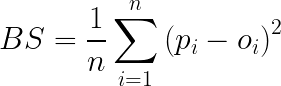Where p is the prediction probability of occurrence of the event, and the term oi is equal to 1 if the event occurred and 0 if not.Let’s take a very quick example to assimilate this concept. Let’s consider the event A=”Tomorrow is a sunny day”.

If you predict that the event A will occur with a probability of 100%, and the event occurs (the next is sunny which means o=1), the Brier score is equal to: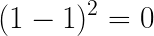This is the lowest value possible. In other words: the best case we can achieve.

If we predicted the same event with the same probability, but the event doesn’t occur, the Brier score in this case is: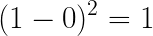Say you predicted that the event A will occur with another probability, let’s say 60%. In case the event doesn’t occur in reality, the Brier Score will be: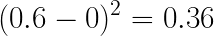As you may have noticed, the Brier score is a distance in the probability domain. Which means: the lower the value of this score, the better the prediction.

A perfect prediction will get a score of 0. The worst score is 1. It’s a synthetic criterion that provides combined information on the accuracy, robustness, and interpretability of the prediction model.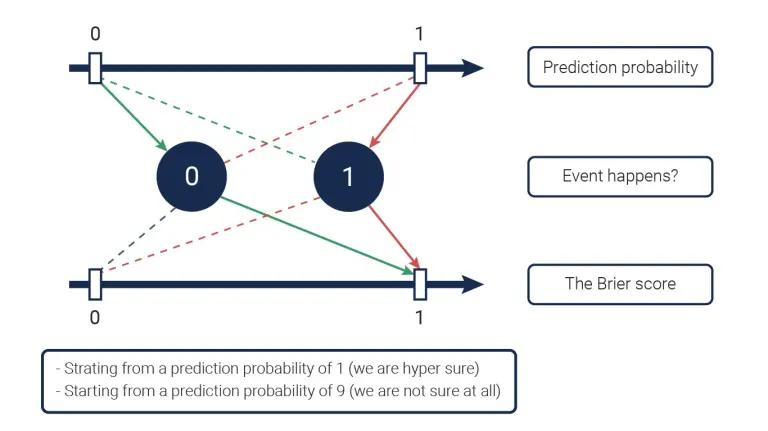Dotted lines represent the worst cases (if the event occurs, the circle is equal to 1).

# # What is probability calibration?

Probability calibration is the post-processing of a model to improve its probability estimate. It helps us compare two models that have the same accuracy or other standard evaluation metrics.

We say that a model is well calibrated when a prediction of a class with confidence p is correct 100 % of the time. To illustrate this calibration effect, let’s consider that you have a model that predicts cancer with a score of 70% for each patient out of 100. If your model is well calibrated, we would have 70 patients with cancer, if it is ill-calibrated, we will have more (or less). Therefore, the difference between these two models:

• A model has an accuracy of 70% with 0.7 confidence in each prediction = well calibrated.
• A model who has an accuracy of 70% with 0.9 confidence in each prediction = ill-calibrated. For a perfect calibration, the relationship between the predicted probability and the fraction of positives follows the given: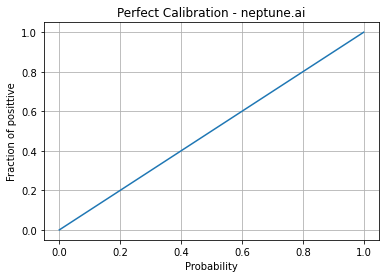The expression of this relationship is given by: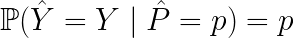The figure above represents the reliability diagram of a model. We can plot it using scikit-learn as below:

’'’python import sklearn from sklearn.calibration import calibration_curve import matplotlib.lines as line import matplotlib.pyplot as plt

x, y=calibration_curve(y_true, y_prob)

plt.plot(x,y) ref = line.Line2D([0, 1], [0, 1], color=’black’) transform = ax.transAxes line.set_transform(transform) ax.add_line(line) fig.suptitle(‘Calibration – Neptune.ai’) ax.set_xlabel(‘Predicted probability’) ax.set_ylabel(‘Fraction of positive’) plt.legend() plt.show() ‘’’

Plotting the reliability curve for multiple models allows us to choose the best model not only based on its accuracy, but on its calibration too. In the figure below, we can eliminate the SVC (0.163) model because it is far from being well calibrated.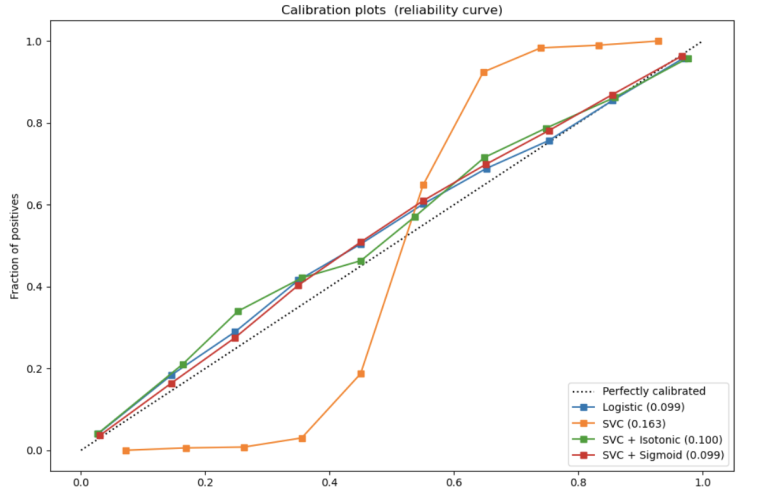If we want a numeric value to check the calibration of our models, we can use the calibration error given theoretically by: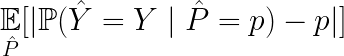## When should you use the Brier score?

Evaluating the performance of a machine learning model is important, but it’s not enough to evaluate the real-world application predictions. We often worry about:

• the model’s confidence in its predictions,
• its error distribution,
• and how probability estimates are made. In such cases, we need to use other performance factors. Brier score is an example.

This type of performance score is specifically used in high-risk applications. This score allows us to not treat the model results as real probabilities, but instead go beyond the raw results and check the model calibration, which is important for avoiding bad decision making or false interpretation.

Rating: